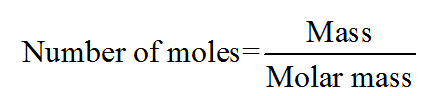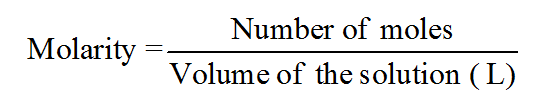# The concentration of sodium chloride in an aqueous solution that is 2.13 M and that has a density of 1.01 g/mL is ________% by mass.

Question
1 views

The concentration of sodium chloride in an aqueous solution that is 2.13 M and that has a density of 1.01 g/mL is ________% by mass.

check_circle

Step 1

The number of moles for a given chemical species refers to the ratio of mass of the species to that of its molar mass. The formula is shown below:Step 2

The molarity of the given chemical solution is related to the number of moles of the solute and the volume of the solution (in liters) as follows:Step 3

The term density can be defined as the measurement which can be used to compare the amount of the matter present in a given object to its volume. An object having much amount of matter in a certain volume will have higher density. The density of a given object can be calculated from the ratio of i...

### Want to see the full answer?

See Solution

#### Want to see this answer and more?

Solutions are written by subject experts who are available 24/7. Questions are typically answered within 1 hour.*

See Solution
*Response times may vary by subject and question.
Tagged in

### Chemistry